Home

# Nilsequences, null-sequences, and multiple correlation sequences

## Abstract

A (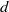$d$ -parameter) basic nilsequence is a sequence of the form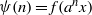$\psi (n)= f({a}^{n} x)$ ,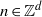$n\in { \mathbb{Z} }^{d}$ , where$x$ is a point of a compact nilmanifold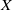$X$ ,$a$ is a translation on$X$ , and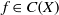$f\in C(X)$ ; a nilsequence is a uniform limit of basic nilsequences. If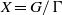$X= G/ \Gamma$ is a compact nilmanifold,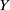$Y$ is a subnilmanifold of$X$ ,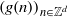$\mathop{(g(n))}\nolimits_{n\in { \mathbb{Z} }^{d} }$ is a polynomial sequence in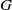$G$ , and$f\in C(X)$ , we show that the sequence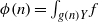$\phi (n)= \int \nolimits \nolimits_{g(n)Y} f$ is the sum of a basic nilsequence and a sequence that converges to zero in uniform density (a null-sequence). We also show that an integral of a family of nilsequences is a nilsequence plus a null-sequence. We deduce that for any invertible finite measure preserving system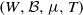$(W, \mathcal{B} , \mu , T)$ , polynomials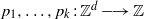${p}_{1} , \ldots , {p}_{k} : { \mathbb{Z} }^{d} \longrightarrow \mathbb{Z}$ , and sets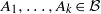${A}_{1} , \ldots , {A}_{k} \in \mathcal{B}$ , the sequence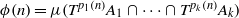$\phi (n)= \mu ({T}^{{p}_{1} (n)} {A}_{1} \cap \cdots \cap {T}^{{p}_{k} (n)} {A}_{k} )$ ,$n\in { \mathbb{Z} }^{d}$ , is the sum of a nilsequence and a null-sequence.

Hide All

# Nilsequences, null-sequences, and multiple correlation sequences

## Metrics

### Full text viewsFull text views reflects the number of PDF downloads, PDFs sent to Google Drive, Dropbox and Kindle and HTML full text views.

Total number of HTML views: 0
Total number of PDF views: 0 *Loading metrics...

### Abstract viewsAbstract views reflect the number of visits to the article landing page.

Total abstract views: 0 *Loading metrics...

* Views captured on Cambridge Core between <date>. This data will be updated every 24 hours.

Usage data cannot currently be displayed.